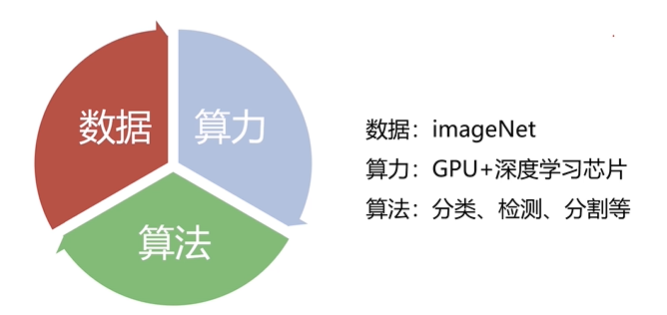2019-04-22 16:01:00 xeonmm1 阅读数 111
• ###### 机器学习&深度学习系统实战！

购买课程后，可扫码进入学习群，获取唐宇迪老师答疑 数学原理推导与案例实战紧密结合，由机器学习经典算法过度到深度学习的世界，结合深度学习两大主流框架Caffe与Tensorflow，选择经典项目实战人脸检测与验证码识别。原理推导，形象解读，案例实战缺一不可！具体课程内容涉及回归算法原理推导、决策树与随机森林、实战样本不均衡数据解决方案、支持向量机、Xgboost集成算法、神经网络基础、神经网络整体架构、卷积神经网络、深度学习框架--Tensorflow实战、案例实战--验证码识别、案例实战--人脸检测。 专属会员卡优惠链接：http://edu.csdn.net/lecturer/1079

39639 人正在学习 去看看 唐宇迪
``````import tensorflow as tf
import numpy as np

# zhonglihao 2019.04

# 训练集
x_train = [[0.0,0.0],[0.0,1.0],[1.0,0.0],[1.0,1.0]]
y_train = [,,,]

# 网络参数 输入向量为2 隐藏层为4个cell 输出1/0单个节点
# zhonglihao: 使用2个隐藏cell也能学 但很容易卡死 所以这里用得多点 训练的时候容易收敛
Input_Node      = 2
Layer1_Node     = 4 # 4颗比较稳
Output_Node     = 1

# 输入输出动态占位符
x   = tf.placeholder(tf.float32, [None, Input_Node])
y_  = tf.placeholder(tf.float32, [None, Output_Node])

# 定义网络参数变量
w1 = tf.Variable(tf.random_normal([Input_Node, Layer1_Node]))
w2 = tf.Variable(tf.random_normal([Layer1_Node, Output_Node]))

b1 = tf.Variable(tf.zeros([Layer1_Node]))
b2 = tf.Variable(tf.zeros([Output_Node]))

# 进行神经网络相乘并使用激活函数sigmoid
Layer1_Output   = tf.sigmoid(tf.matmul(x,w1)+b1)
pred            = tf.sigmoid(tf.matmul(Layer1_Output,w2)+b2)

# 计算损失，这里使用方差
cost = tf.reduce_mean(tf.square(y_ - pred))

# 学习率
learning_rate = 0.01

# 优化器

# 全局变量初始化
init = tf.global_variables_initializer()

with tf.Session() as sess:
sess.run(init)
# 自定义训练轮数
max_step = 10000
for i in range(max_step + 1):
sess.run(train_step, feed_dict = {x: x_train, y_: y_train})
loss = sess.run(cost, feed_dict = {x: x_train, y_: y_train})
if (i % 100 == 0):
print(sess.run(pred, feed_dict = {x: x_train}))
print('end')``````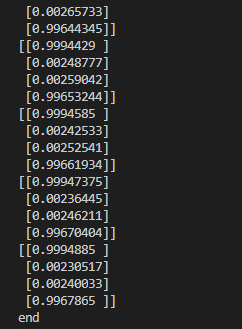2019-09-03 10:14:55 joker_shy 阅读数 868
• ###### 机器学习&深度学习系统实战！

购买课程后，可扫码进入学习群，获取唐宇迪老师答疑 数学原理推导与案例实战紧密结合，由机器学习经典算法过度到深度学习的世界，结合深度学习两大主流框架Caffe与Tensorflow，选择经典项目实战人脸检测与验证码识别。原理推导，形象解读，案例实战缺一不可！具体课程内容涉及回归算法原理推导、决策树与随机森林、实战样本不均衡数据解决方案、支持向量机、Xgboost集成算法、神经网络基础、神经网络整体架构、卷积神经网络、深度学习框架--Tensorflow实战、案例实战--验证码识别、案例实战--人脸检测。 专属会员卡优惠链接：http://edu.csdn.net/lecturer/1079

39639 人正在学习 去看看 唐宇迪

## 数据如下

``````x = [[0, 0], [1, 0], [0, 1], [1, 1]]
y = [, , , ]
``````

## 代码实现

``````import tensorflow as tf
import matplotlib.pyplot as plt

tf.set_random_seed(33)

x = [[0, 0], [1, 0], [0, 1], [1, 1]]
y = [, , , ]

X = tf.placeholder(tf.float32, shape=[None, 2])
Y = tf.placeholder(tf.float32, shape=[None, 1])

w1 = tf.Variable(tf.random_normal([2, 3]))
b1 = tf.Variable(tf.random_normal())
w2 = tf.Variable(tf.random_normal([3, 1]))
b2 = tf.Variable(tf.random_normal())

z1 = tf.matmul(X, w1) + b1
a1 = tf.sigmoid(z1)
z2 = tf.matmul(a1, w2) + b2
a2 = tf.sigmoid(z2)

cost = -tf.reduce_mean(Y * tf.log(a2) + (1 - Y) * tf.log(1 - a2))
cost_history = []

m = tf.cast(tf.shape(X), tf.float32)
dz2 = a2 - Y
dw2 = tf.matmul(tf.transpose(a1), dz2) / m
db2 = tf.reduce_mean(dz2, axis=0)

da1 = tf.matmul(dz2, tf.transpose(w2))
dz1 = da1 * a1 * (1 - a1)
dw1 = tf.matmul(tf.transpose(X), dz1) / m
db1 = tf.reduce_mean(dz1, axis=0)

learning_rate = 3.8 * 10e-2
updata = [
tf.assign(w2, w2 - learning_rate * dw2),
tf.assign(b2, b2 - learning_rate * db2),
tf.assign(w1, w1 - learning_rate * dw1),
tf.assign(b1, b1 - learning_rate * db1)
]

with tf.Session() as sess:
sess.run(tf.global_variables_initializer())
for step in range(10001):
cost_var , _ = sess.run([cost, updata], feed_dict={X: x, Y: y})
if step % 500 == 0:
print('Step', step, 'Cost', cost_var)
cost_history.append(cost_var)

predict = sess.run(a2, feed_dict={X: x})

print('预测值为', predict)

plt.plot(cost_history[1:])
plt.show()

``````

## 结果如下

``````Step 0 Cost 0.9873838
Step 500 Cost 0.36180282
Step 1000 Cost 0.059805408
Step 1500 Cost 0.027795581
Step 2000 Cost 0.017842356
Step 2500 Cost 0.013083072
Step 3000 Cost 0.010309634
Step 3500 Cost 0.008498434
Step 4000 Cost 0.00722454
Step 4500 Cost 0.006280598
Step 5000 Cost 0.005553428
Step 5500 Cost 0.0049763597
Step 6000 Cost 0.0045073307
Step 6500 Cost 0.004118765
Step 7000 Cost 0.00379156
Step 7500 Cost 0.0035123003
Step 8000 Cost 0.0032712156
Step 8500 Cost 0.003060991
Step 9000 Cost 0.0028760713
Step 9500 Cost 0.0027121357
Step 10000 Cost 0.002565823

[9.9655342e-01]
[9.9778229e-01]
[3.7732117e-03]]

``````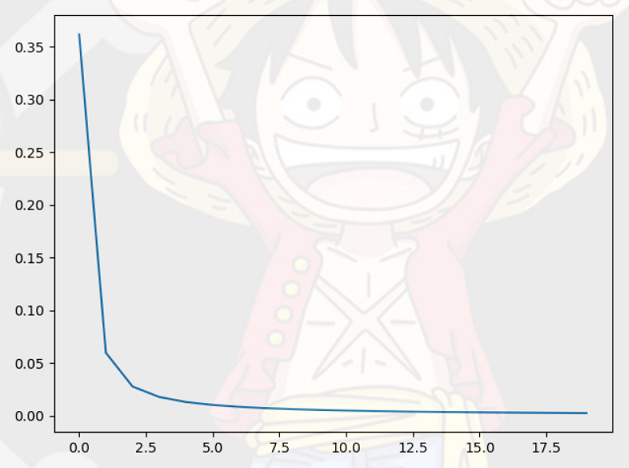2018-09-11 20:14:55 u013539952 阅读数 620
• ###### 机器学习&深度学习系统实战！

购买课程后，可扫码进入学习群，获取唐宇迪老师答疑 数学原理推导与案例实战紧密结合，由机器学习经典算法过度到深度学习的世界，结合深度学习两大主流框架Caffe与Tensorflow，选择经典项目实战人脸检测与验证码识别。原理推导，形象解读，案例实战缺一不可！具体课程内容涉及回归算法原理推导、决策树与随机森林、实战样本不均衡数据解决方案、支持向量机、Xgboost集成算法、神经网络基础、神经网络整体架构、卷积神经网络、深度学习框架--Tensorflow实战、案例实战--验证码识别、案例实战--人脸检测。 专属会员卡优惠链接：http://edu.csdn.net/lecturer/1079

39639 人正在学习 去看看 唐宇迪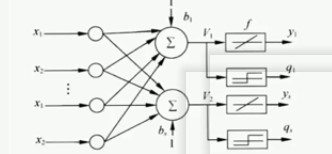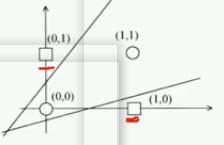线性神经网络的办法：对神经元加入非线性输入，引入非线性成分，使等效的输入维度变大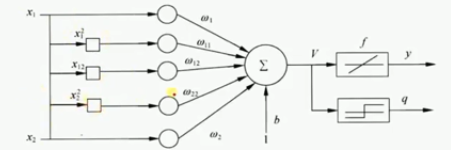如图，输入x1，x2分别为1，0。再加上引入的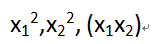一共5个输入，y为线性激活函数，用来训练模型数据，输出仍用sign，仍为(0,1)两类。

1.导入包

``````import numpy as np
import matplotlib.pyplot as plt
``````

2.输入数据

5个输入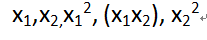在加上偏置值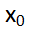设为1，则一共输入为6个数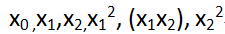``X = np.array([[1,0,0,0,0,0],[1,0,1,0,0,1],[1,1,0,1,0,0],[1,1,1,1,1,1]])``

3.标签  0 0 为 -1 10 为1 01 为1 11 为-1

``Y = np.array([-1,1,1,-1])``

4.权值初始化 6个权值 初始化为6 取值范围 -1,1

``````W = (np.random.random(6)-0.5)*2
print(W)``````

5.设置学习率等数据

``````#学习率
lr = 0.11

#计算迭代次数1
n = 0

#神经网络输出
O = 0``````

6.更新权值函数

``````# 更新权值函数
def update():
global X,Y,W,lr,n
n+=1
#输出 激活函数改变
#O = np.sign(np.dot(X,W.T))
O = np.dot(X,W.T)
#计算权值的改变   求平均 X的行
W_C = lr*((Y-O.T).dot(X))/int(X.shape)
W = W + W_C

for _ in range(1000):
#更新权值函数
update()

#无论是否收敛 都循环1000次``````

1. 和期望值比误差小
2. 权值的改变量小
3. 进行多次循环

这里是进行1000次循环来使函数收敛

7.设置正负样本

``````#正样本
x1 = [0,1]
y1 = [1,0]
#负样本
x2 = [0,1]
y2 = [0,1]``````

8.画图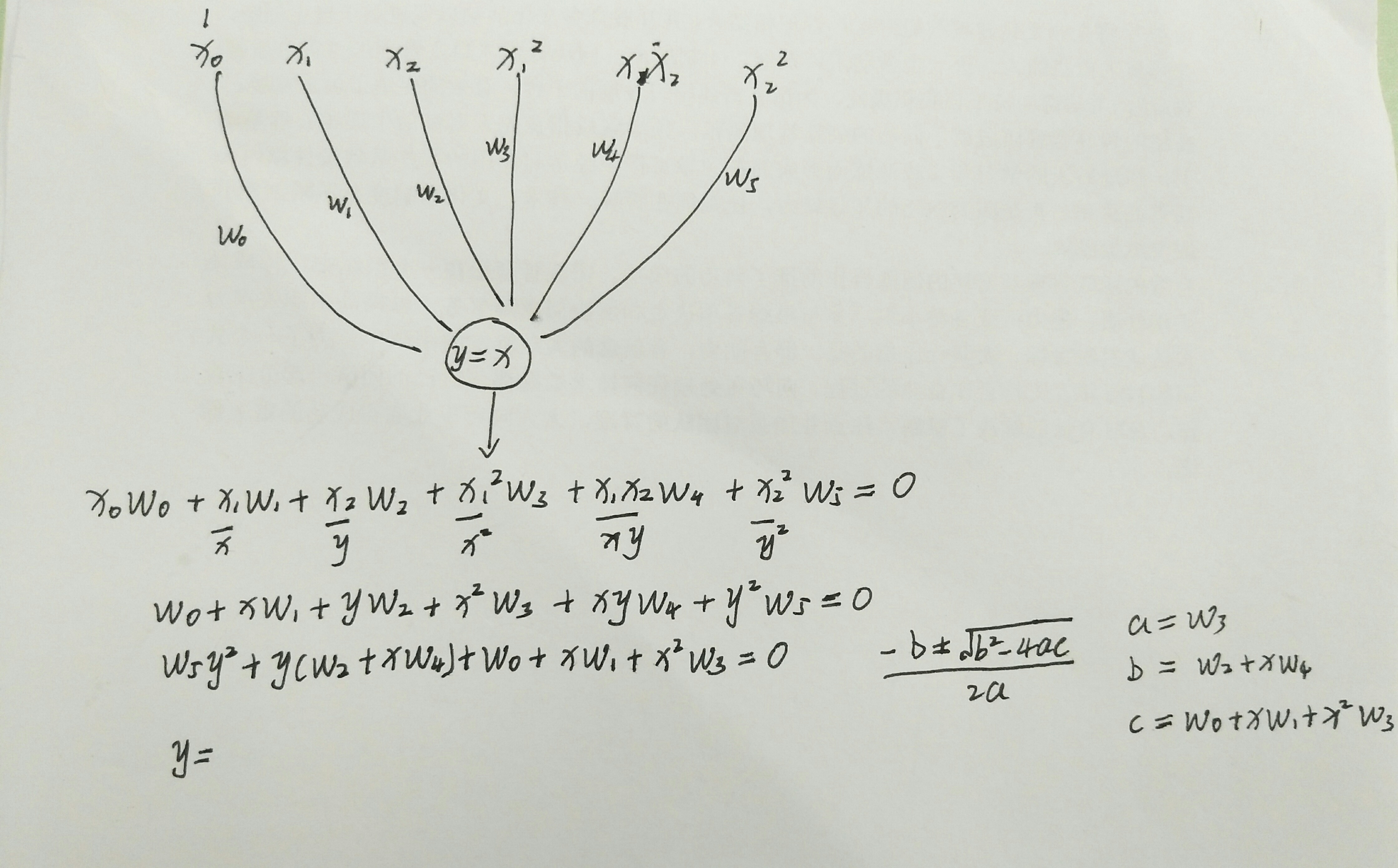``````#划线
def calculate(x,root):
a = W
b = W + x*W
c = W + x*W + x*x*W
if root==1:
return (-b+np.sqrt(b*b-4*a*c))/(2*a)
if root == 2:
return (-b-np.sqrt(b*b-4*a*c))/(2*a)

#取-1到2的点
xdata = np.linspace(-1,2)
#画图
plt.figure()
#有两个值 画两条线
plt.plot(xdata,calculate(xdata,1),'r')
plt.plot(xdata,calculate(xdata,2),'r')

#正样本 蓝色表示
plt.plot(x1,y1,'bo')
#负样本 黄色表示
plt.plot(x2,y2,'yo')
plt.show()``````

9.查看输出数据

``````# X 输入数据 W.T迭代1000次
O = np.dot(X,W.T)
print(O)``````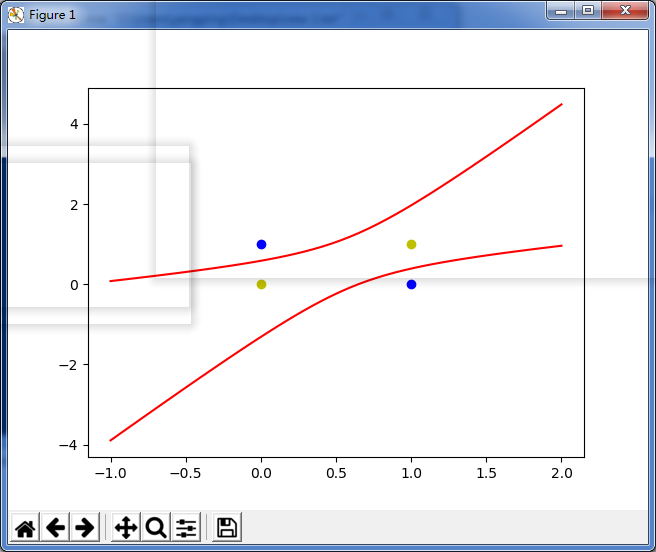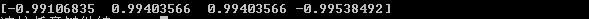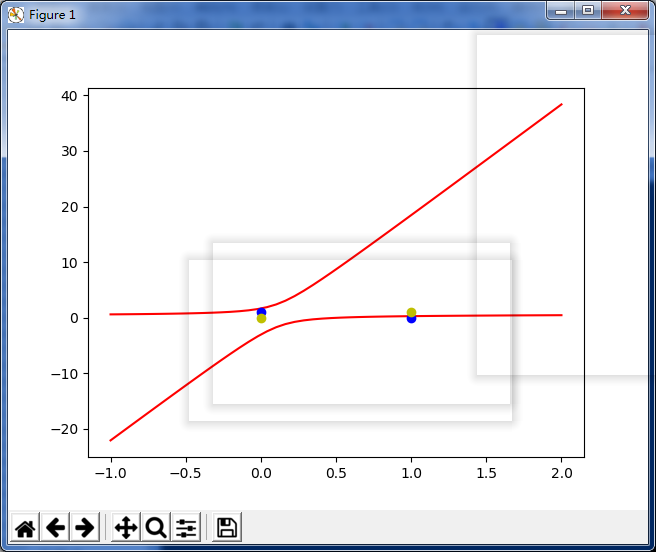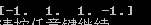2018-05-30 17:25:09 fengzhongluoleidehua 阅读数 1404
• ###### 机器学习&深度学习系统实战！

购买课程后，可扫码进入学习群，获取唐宇迪老师答疑 数学原理推导与案例实战紧密结合，由机器学习经典算法过度到深度学习的世界，结合深度学习两大主流框架Caffe与Tensorflow，选择经典项目实战人脸检测与验证码识别。原理推导，形象解读，案例实战缺一不可！具体课程内容涉及回归算法原理推导、决策树与随机森林、实战样本不均衡数据解决方案、支持向量机、Xgboost集成算法、神经网络基础、神经网络整体架构、卷积神经网络、深度学习框架--Tensorflow实战、案例实战--验证码识别、案例实战--人脸检测。 专属会员卡优惠链接：http://edu.csdn.net/lecturer/1079

39639 人正在学习 去看看 唐宇迪

https://blog.csdn.net/hjimce/article/details/50573444

https://blog.csdn.net/column/details/ym-alanyannick.html

2019-08-20 22:31:06 weixin_43216249 阅读数 84
• ###### 机器学习&深度学习系统实战！

购买课程后，可扫码进入学习群，获取唐宇迪老师答疑 数学原理推导与案例实战紧密结合，由机器学习经典算法过度到深度学习的世界，结合深度学习两大主流框架Caffe与Tensorflow，选择经典项目实战人脸检测与验证码识别。原理推导，形象解读，案例实战缺一不可！具体课程内容涉及回归算法原理推导、决策树与随机森林、实战样本不均衡数据解决方案、支持向量机、Xgboost集成算法、神经网络基础、神经网络整体架构、卷积神经网络、深度学习框架--Tensorflow实战、案例实战--验证码识别、案例实战--人脸检测。 专属会员卡优惠链接：http://edu.csdn.net/lecturer/1079

39639 人正在学习 去看看 唐宇迪

# 深度学习基础

## 深度学习发展历程

### 三起两落的深度学习：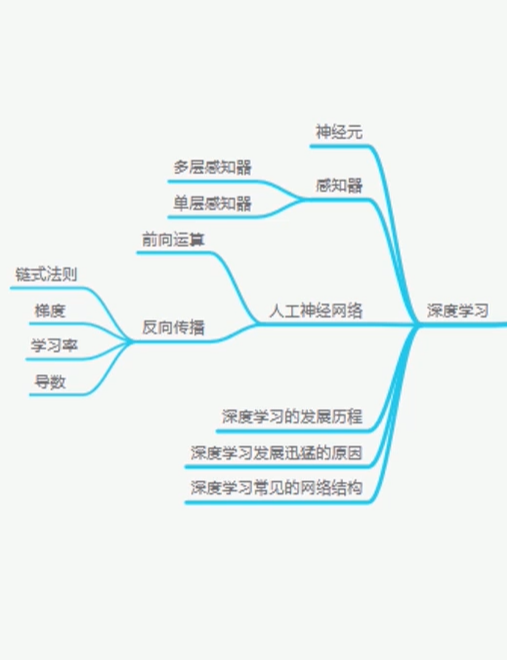1943年，由一位心理学家和一位数学家共同提出人工神经网络的基本概念，不过在当时并没有得到大众的重视。直到1957年感知器模型的提出，使得神经网络可以解决一些常规的线性分类和线性回归的任务，这引发了AI研究的热潮，但是在之后的研究中发现，它无法解决简单的异或问题（XOR),因此迎来了第一次低潮。虽然在1980年多层感知器解决了单层感知器的问题，但是对其网络参数的计算问题却成为了一个难点。但是在1986时，反向传播算法的提出解决了对少数层神经网络训练的问题，虽然依然存在一些问题，但是受限于但是的条件，通过反向传播能够简单，低效的对多层感知器进行学习，这个时候也就迎来了第二次高潮。同时，该算法的也存在缺陷，主要体现在两个方面，随着深度的加深，容易产生梯度的消失或者梯度爆炸，使得网络的参数没有得到实施的解决。除此之外呢，该算法容易陷入局部最优的问题，因此呢，便迎来了第二次低谷。而在1988年时，尽管当时提出了卷积神经网络，但是却并没有得到过多的重视。直到2006年，提出了深度学习，得益于时代的发展，人工神经网再次得到了发展，尤其在2012年，AlexNet算法的提出，引起瞩目，在此之后，各种优质算法层出不穷，作用于各个方面。

## 什么是人工神经网络

### 什么是感知器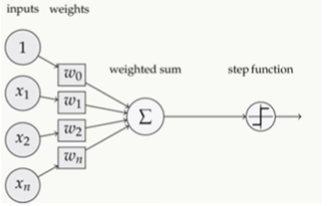### 什么是深度学习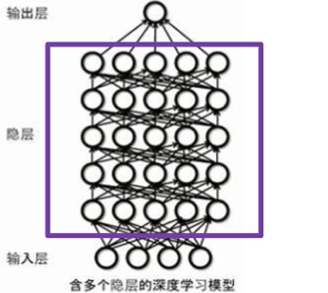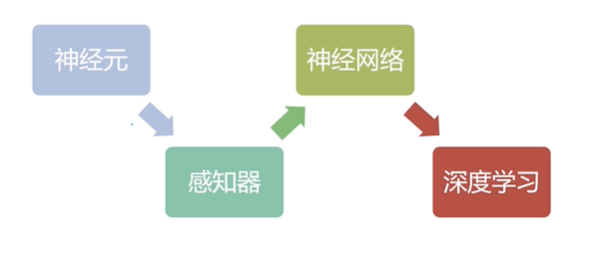## 深度学习中的核心知识

### 前向运算与反向运算：

#### 前向运算：

##### 同时，这里引入了监督的概念：

2.函数中的相关参数的值分别是什么。

#### 什么是导数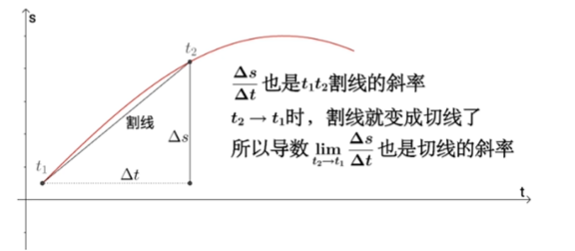##### 什么是方向导数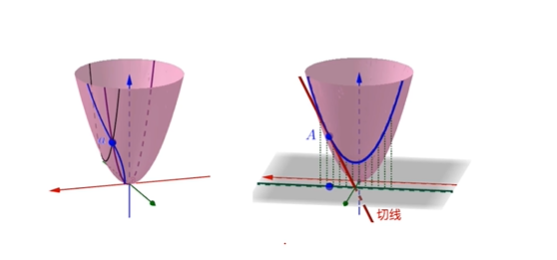##### 什么是偏导数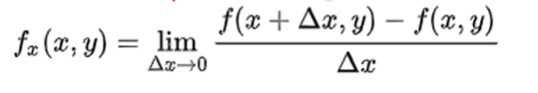##### 什么是梯度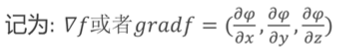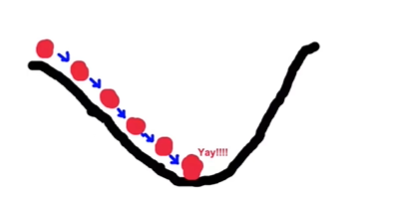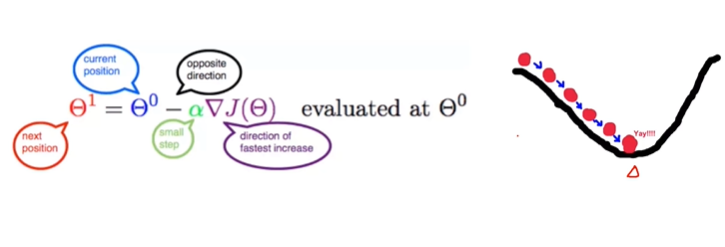1、求梯度，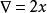2、向梯度相反的方向移动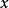，如下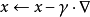，其中，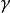为步长。如果步长足够小，则可以保证每一次迭代都在减小，但可能导致收敛太慢，如果步长太大，则不能保证每一次迭代都减少，也不能保证收敛。
3、循环迭代步骤2，直到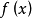的值变化到使得 在两次迭代之间的差值足够小，比如0.00000001，也就是说，直到两次迭代计算出来的基本没有变化，则说明此时已经达到局部最小值了。
4、此时，输出，这个就是使得函数在这里插入图片描述最小时的的取值 。

#### 局部最优解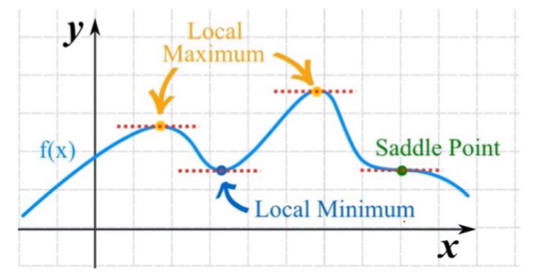#### 迭代过程以及参数优化概念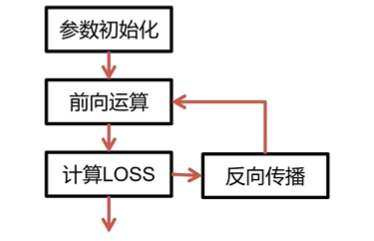###### 参数更新：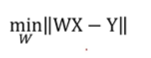W为参数，X为输入值，WX则为预测值，Y为标签值。因此||WX-Y||得出的结果即为Loss，而在不断地优化地过程中则是不断缩小Loss，而不断更新参数。

## 深度学习发展迅猛的原因